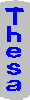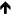Map Index Random Help Topics## Topic: mathematics by proofs and refutations

topics > philosophy > Group: philosophy of mathematics

Topic:
language and life as a game
Topic:
limitations of formalism
Topic:
mathematical proof
Topic:
mathematical proof as a social process
Topic:
problems with analytic truth
Topic:

#### Summary

Mathematics by proofs and refutations is Lakatos's analysis of the history of Euler's relation, V-E+F=2. It is related to Feynman's "Babylonian mathematics", and to mathematics as a social process.

Euler's relation has a long and complicated history. Many formulations have been tried, only to be refuted and replaced by another formulation. Lakatos sees this as true of mathematics in general. Writing a proof makes explicit the assumptions behind an idea. These assumptions may lead to counter-examples, which then cause generalization and refinement of the original theorem. (cbb 4/94)

Subtopic: proofs may contain flaws, speculation, heuristicsQuote: proofs and refutations--a proof can be respectable without being flawless; Seidel 1847, c.f. Hegel and Popper [»lakaI_1976] Quote: informal mathematics does not grow by indubitable theorems but by speculation, proof, and refutation [»lakaI_1976] Quote: mathematicians use a situational logic that is neither formal, mechanistic, nor irrational blind guessing [»lakaI_1976] Quote: mathematical heuristic is like scientific heuristic but with different conjectures, explanations, and counterexamples [»lakaI_1976]

Subtopic: proof as thought-experiment -- conjectureQuote: proof is a thought-experiment that decomposes a conjecture into lemmas that belong to a body of knowledge [»lakaI_1976] Quote: Cauchy's proof of Euler's relation used conjectures about planar maps, triangulations, and the effect of removing triangles [»lakaI_1976] Quote: the students guessed that Euler's relation held for all polyhedron, testing didn't falsify; so attempt a proof [»lakaI_1976] Quote: naive conjectures are arrived at by trial and error; e.g., used in building a table for Euler's relation [»lakaI_1976] Quote: can deductively guess Euler's relation from V=E for polygons; no need for facts and data [»lakaI_1976] Quote: proofs and refutations--to improve a conjecture may need many proofs; thus expanding the conjecture's domain [»lakaI_1976]

Subtopic: method of proof and refutationQuote: method of proof and refutation: 1) inspect proof for non-trivial lemmas and seek local and global counterexamples [»lakaI_1976] Quote: method of proof and refutations: 2) if a global counterexample, make the hidden lemma explicit and a condition of the conjecture [»lakaI_1976] Quote: should prove self-evident conjectures since they may hinge on a dubious lemma whose refutation leads to a global refutation [»lakaI_1976] Quote: method of proof and refutations: 4) if a truly local counterexample, try to replace the refuted lemma and increase the theorem's content [»lakaI_1976] Quote: lemma-incorporation--modify a conjecture by analyzing why the proof's components failed for a counterexample; i.e., proof and refutations [»lakaI_1976] Quote: one should scrutinize a proof to see if any assumptions can be removed; use counterexamples to show the necessary ones [»lakaI_1976] Quote: the process of lemma-incorporation yields an infinite regress unless proof is a game or there are trivially true lemmas [»lakaI_1976] Quote: lemma-incorporation results in a theorem that is defined by the proof [»lakaI_1976]

Subtopic: monster-barring -- restricted domainQuote: if you have too much respect for facts that refute your conjectures, you may miss conjectures that can be tested with thought-experiments [»lakaI_1976] Quote: monster-barring: since the theorem was proved, counter-examples can be barred; e.g., a pair of nested cubes is not a polyhedron [»lakaI_1976] Quote: a theorem applies to a restricted domain; so counter-examples are exceptions to the conjecture instead of monsters [»lakaI_1976] Quote: monster-adjustment: the 'urchin' counterexample was actually a misinterpretation of what are edges, faces, and vertices [»lakaI_1976]

Subtopic: seeking deeper theoremsQuote: proofs and refutations: 5) if you have counterexamples, try to find, by deductive guessing, a deeper theorem that handles them [»lakaI_1976] Quote: as well as certainty, want finality in a theorem; e.g., one that covers all polyhedra that satisfy Euler's relation [»lakaI_1976] Quote: proof-generated concepts replace the original naive concepts completely; very different [»lakaI_1976] Quote: chain spaces is the proof-generated concept that yields an indubitable formulation of Euler's theorem [»lakaI_1976] Quote: mathematicians want to improve their conjectures without refuting them; yields a monotonous increase of truth [»lakaI_1976]

Subtopic: teaching via proofs and refutationsQuote: should teach mathematics by showing the development of proof-generated concepts from naive conjectures; dispels mysticism of mathematics [»lakaI_1976]

Subtopic: Babylonian mathematics -- experimentalQuote: physicists do Babylonian mathematics because different formulations of the same law give different clues for related laws [»feynR_1965] Quote: unlike the Euclidean method, the Babylonian idea is to remember enough to work something out or reconstruct what is needed [»feynR_1965] Quote: a derivation or proof has only probabilitistic validity; mathematics is somewhat like experimental sciences with repeatable experiments and widespread usage [»daviPJ3_1972]

Related TopicsTopic: language and life as a game (30 items)
Topic: limitations of formalism (93 items)
Topic: mathematical proof (23 items)
Topic: mathematical proof as a social process (14 items)
Topic: problems with analytic truth (20 items)
Topic: scientific paradigms and research programs
(30 items)

Updated barberCB 8/04